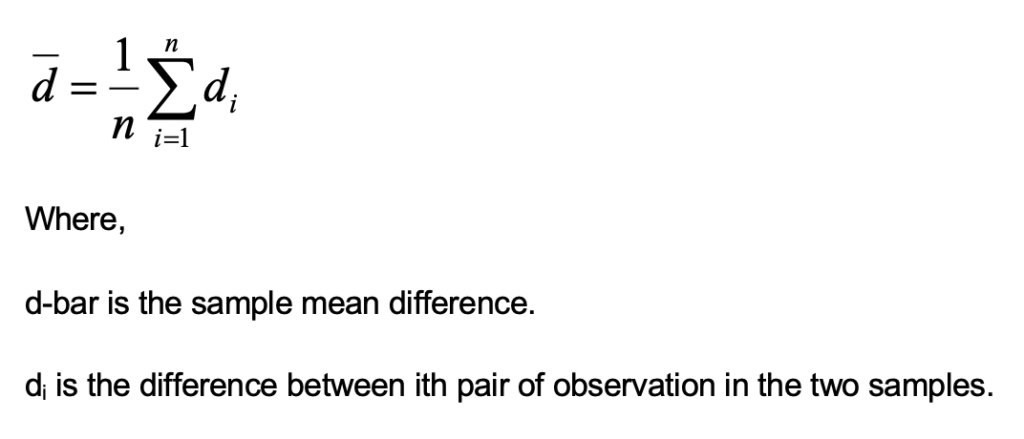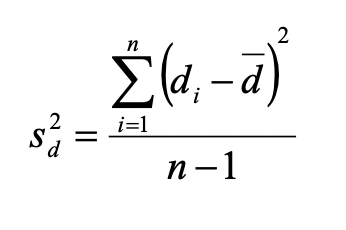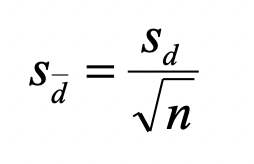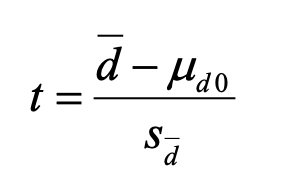# Paired Comparision Tests - Mean Differences When Populations are Not Independent

In the above cases, we assumed that the samples are independent. However, sometimes the samples may not be independent.

If the samples are not independent, a test of mean difference is done using paired observations. Such a test is called paired comparison test.

1. H0: µd = µd0 versus HA: µd ≠ µd0
2. H0: µd ≤ µd0 versus HA: µd > µd0
3. H0: µd ≥ µd0 versus HA: µd < µd0

In the first hypothesis we want to test if the mean of the differences in pairs is zero.

µd is the mean of the population of paired differences.

µd0 is the hypothesized value of mean of paired differences. This is usually assumed to be zero.

### Calculating t-statistic

To calculate the t-statistic, we first need to find the sample mean difference:The sample variance is:The standard deviation of the mean is:The test statistic, with n – 1 df is:Get our Data Science for Finance Bundle for just $29$51.